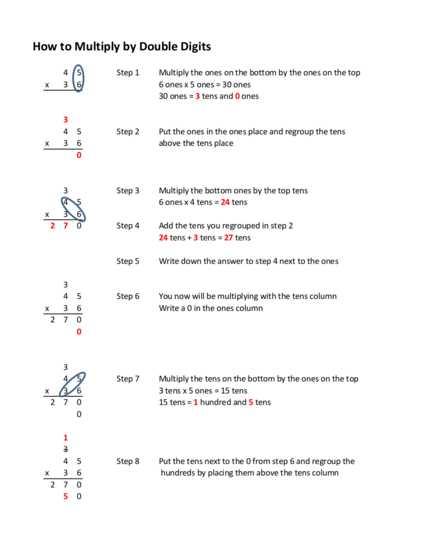# How to Multiply a Number by a Two-Digit NumberContributed by:Learn the steps needed to multiply a number by a two-digit number.
1. How to Multiply by Double Digits
4 5 Step 1 Multiply the ones on the bottom by the ones on the top
x 3 6 6 ones x 5 ones = 30 ones
30 ones = 3 tens and 0 ones
3
4 5 Step 2 Put the ones in the ones place and regroup the tens
x 3 6 above the tens place
0
3 Step 3 Multiply the bottom ones by the top tens
4 5 6 ones x 4 tens = 24 tens
x 3 6
2 7 0 Step 4 Add the tens you regrouped in step 2
24 tens + 3 tens = 27 tens
Step 5 Write down the answer to step 4 next to the ones
3
4 5 Step 6 You now will be multiplying with the tens column
x 3 6 Write a 0 in the ones column
2 7 0
0
3
4 5 Step 7 Multiply the tens on the bottom by the ones on the top
x 3 6 3 tens x 5 ones = 15 tens
2 7 0 15 tens = 1 hundred and 5 tens
0
1
3
4 5 Step 8 Put the tens next to the 0 from step 6 and regroup the
x 3 6 hundreds by placing them above the tens column
2 7 0
5 0
2. 1
3
4 5 Step 9 Multiply the tens on the bottom by the tens on the top
x 3 6 3 tens x 4 tens = 12 hundreds
2 7 0
5 0 Step 10 Add the regrouped amount from step 8
12 hundreds + 1 hundred = 13 hundreds
1
3
4 5 Step 11 Write down the answer to step 10
x 3 6
2 7 0
1 3 5 0
1
3
4 5 Step 12 Add the two numbers
x 3 6 0 ones + 0 ones = 0 ones
1
2 7 0
+ 1 3 5 0 7 tens + 5 tens = 12 tens
1 6 2 0 12 tens = 1 hundred and 2 tens
Write the 2 in the tens place and regroup the 1 hundred
1 hundred + 2 hundreds + 3 hundreds = 6 hundreds
0 thousands + 1 thousand = 1 thousand